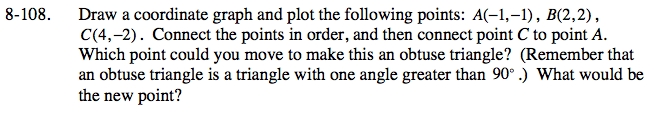Home > ACC7 > Chapter cc28 > Lesson cc28.3.4 > Problem8-108

8-108.

8‑108. Draw a coordinate graph and plot the following points: A(−1, −1), B(2 , 2), C(4,−2). Connect the points in order, and then connect point C to point A. Which point could you move to make this an obtuse triangle? (Remember that an obtuse triangle is a triangle with one angle greater than 90°.) What would be the new point? 8-108 HW eTool (Desmos). Homework Help ✎Use the eTool below to help solve the problem.
Click the link at right for the full version of the eTool: CC2 8-108 HW eTool